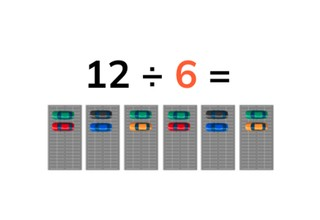Solving the division table of 6

# Solving the division table of 6

Students learn to solve the division table of 6.8,000 schools use Gynzy92,000 teachers use Gynzy1,600,000 students use Gynzy

## General

The students learn to solve the division table of 6.

## Standards

CCSS.Math.Content.3.OA.B.5/6

## Learning objective

Students will be able to solve the division table of 6.

## Introduction

You ask students to volunteer to come up and calculate the jumps of 6 that the monkey takes on the board. You do this both forwards and backwards.

## Instruction

First you discuss the importance of calculating division problems. Next you explain that in a division problem you have a certain amount in each group and that in the division table of 6 you divide an amount by 6. For this you show an example with 18 cars, that you are going to divide evenly between 6 parking lots. From here you can use the bar on the bottom right to navigate through the lesson (visual, abstract, story) in and order that best suits your learning goals. Otherwise you continue with the pages in order and go on to problems with visual support. Here you explain how you solve a division problem by showing how a division problem works with images of cars that need to be divided between parking lots, next you practice by dividing a number of slices of bread between 6 cutting boards. After this you show images and the students must come up with the problem on their own that matches each image. Next you show an image and ask a student to volunteer to divide the cows between the farmers and the people between the houses. After this you have the students solve division problems on their own using an image. Next you discuss the abstract problems. First you show what the division table of 6 looks like when you divide the number 6 evenly. Then you show division problems with 6. Next the students practice with these insights. Then you walk through the steps to solving a story problem. The students can click on the speaker to listen to the story.

Check whether the students can solve problems from the division table of 6 by asking them the following questions:
- What does the problem 12 ÷ 6 mean? Can you name an example?
- Can you flip the numbers of a division problem?

## Quiz

The students test their understanding of solving the division table of 6 through ten exercises. In some exercises there is visual support for the division problems, others are abstract problems and other exercises are story problems.

## Closing

You have the students solve another problem with visual support, then another abstract problem and one more story problem. To close you can play tag. A student says the name of another student and a problem from the division table of 6. They then count to three and then run after the student that they named to tag them. When the student gives the answer on time the person who was "it" has to ask another student another question, and if the second student fails to give the correct answer in time then they become "it".

## Teaching tips

You can have the students make division problems with concrete materials by making groups of 6 with them, or they can practice problems on a number line.

## Instruction materials

MAB blocks or another concrete material for each pair of students

### The online teaching platform for interactive whiteboards and displays in schools

• Save time building lessons

• Manage the classroom more efficiently

• Increase student engagement